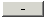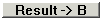# Matrix Subtraction CalGraph will subtract two matrices.

Enter the first matrix on the left and the second matrix on the right.

Now click on the.

Your answer appears at the bottom middle of the window.

You can only subtract matrices if they are the same size, that is, both have the same number of rows and both have the same number of columns. You can subtract two 3 by 3 matrices; but not a 2 by 3 from a 3 by 3.

If you have more matrix arithmetic to do, useto move the answer to the left side

(orto move the matrix to the right side).